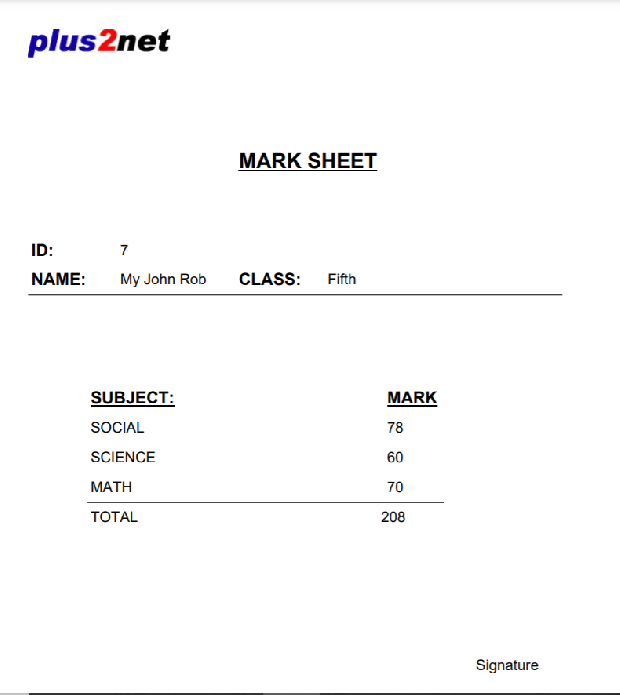# Display single record in a PDF page using variable from Query string

We can generate PDF page by taking data from the query string. This way we will pass variables to our script and using that we will generate our PDF page.

Generating PDF document using record from MySQL database with unique id in PHP using FPDF class

## Example

We will create a page to show mark obtained by each student by using a single PHP script. Our PHP script will receive student ID from query sting and generate the mark sheet of the student by taking data from the table.

## Displaying list of students in a pdf document.

We will be using one similar student list and then on clicking on the name column of the record we will display the Mark sheet of the student. The link we will click will pass the student number or ID to our script. ( Details on adding QR code is explained below )
IDNameWith QR code
2 Max Ruin Max Ruin
3 Arnold Arnold
4 Krish Star Krish Star
5 John Mike John Mike
6 Alex John Alex John
7 My John Rob My John Rob
8 Asruid Asruid
9 Tes Qry Tes Qry
10 Big John Big John```<?Php
// connection to database
require "config.php";
require('fpdf.php');
\$pdf = new FPDF();
//collect student id from URL
\$id=\$_GET['id'];
if(!is_numeric(\$id)){
exit;
}
\$q="select id,name,class,social,science,math,
(social + science + math) AS total from student3 where id=:id";
\$count=\$dbo->prepare(\$q);
\$count->bindParam(":id",\$id,PDO::PARAM_INT,1);

if(\$count->execute()){
\$row = \$count->fetch(PDO::FETCH_OBJ);
\$pdf->Image(\$QR,150,5,50,50,'PNG');

\$pdf->Image('../images/top2.jpg',10,10);
\$pdf->SetFont('Arial','BU',20);
\$pdf->SetXY(80,50);
\$pdf->Cell(30,10,'MARK SHEET',0,0,'L',false);
\$pdf->SetY(80);
\$pdf->SetFont('Arial','B',16);
\$pdf->Cell(30,10,'ID:',0,0,'L',false);
\$pdf->SetFont('Arial','',14);
\$pdf->Cell(40,10,\$row->id,0,1,'L',false);

\$pdf->SetFont('Arial','B',16);
\$pdf->Cell(30,10,'NAME:',0,0,'L',false);
\$pdf->SetFont('Arial','',14);
\$pdf->Cell(40,10,\$row->name,0,0,'L',false);

\$pdf->SetFont('Arial','B',16);
\$pdf->Cell(30,10,'CLASS:',0,0,'L',false);
\$pdf->SetFont('Arial','',14);
\$pdf->Cell(40,10,\$row->class,0,0,'L',false);

\$pdf->SetY(110);
\$pdf->Line(10,100,190,100);
\$pdf->SetXY(30,130);
\$pdf->SetFont('Arial','UB',16);
\$pdf->Cell(100,10,'SUBJECT:',0,0,'L',false);
\$pdf->Cell(50,10,'MARK',0,1,'L',false);
\$pdf->SetFont('Arial','',14);
\$pdf->SetX(30);
\$pdf->Cell(100,10,'SOCIAL',0,0,'L',false);
\$pdf->Cell(50,10,\$row->social,0,1,'L',false);

\$pdf->SetX(30);
\$pdf->Cell(100,10,'SCIENCE',0,0,'L',false);
\$pdf->Cell(50,10,\$row->science,0,1,'L',false);

\$pdf->SetX(30);
\$pdf->Cell(100,10,'MATH',0,0,'L',false);
\$pdf->Cell(50,10,\$row->math,0,1,'L',false);

\$pdf->Line(30,170,150,170);

\$pdf->SetX(30);
\$pdf->Cell(98,10,'TOTAL',0,0,'L',false);
\$pdf->Cell(50,10,\$row->total,0,1,'L',false);

\$pdf->SetXY(160,220);
\$pdf->Cell(50,10,'Signature',0,1,'L',false);
\$pdf->Output();
}else{
print_r(\$dbo->errorInfo());
}
?>```

Inside the zip file you can open index1.php file to list all students in PDF , click the mark column to display the mark sheet of individual student ( index-pdf-mark.php is included inside the zip file.)

Check the readme.txt file for more detail.

## Adding QR code to PDF document.

We can generate QR code by using the student id and the same can be inserted to the generated PDF document.
We will generate one QR code image by using Google chart API and same image we will insert to our document.
Here is the code to create the image ( QR code ) and add the same to our document.
``````\$QR="https://chart.googleapis.com/chart?chs=50x50&cht=qr&chl=\$row->id";
\$pdf->Image(\$QR,150,5,50,50,'PNG');``````

Subscribe to our YouTube Channel here

## Subscribe

* indicates required
Subscribe to plus2netplus2net.com